# RSoft Application Gallery Note: Monte Carlo Analysis: Uncertainties in Q and BER

## Tool Used: OptSim

This example discusses confidence intervals for Q and BER estimation due to Monte Carlo analysis.

The figure below depicts the layout for this example: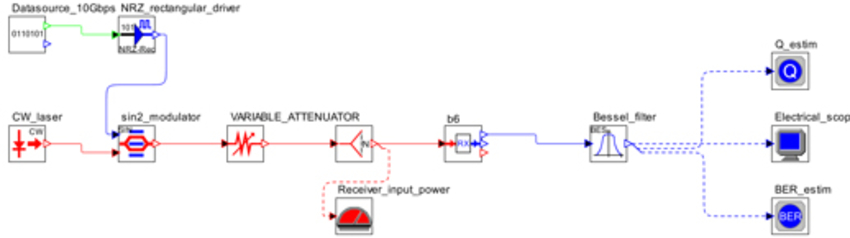When 32 bits are simulated and the Q is estimated, the plot of Q vs. Received Power is not even monotonic: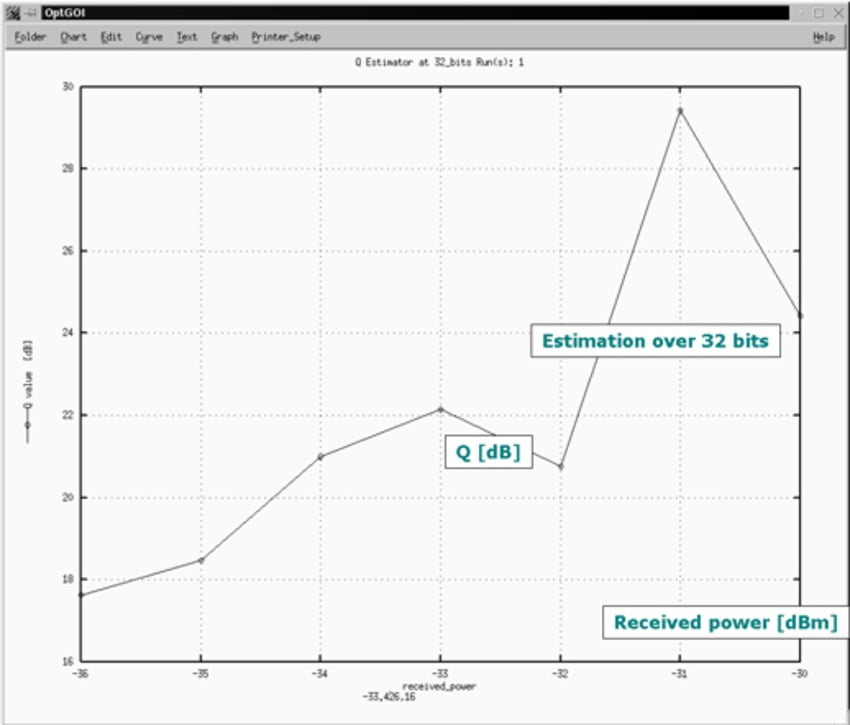When 512 bits are simulated, the Q-estimation plor vs the received power is smoother and monotonic: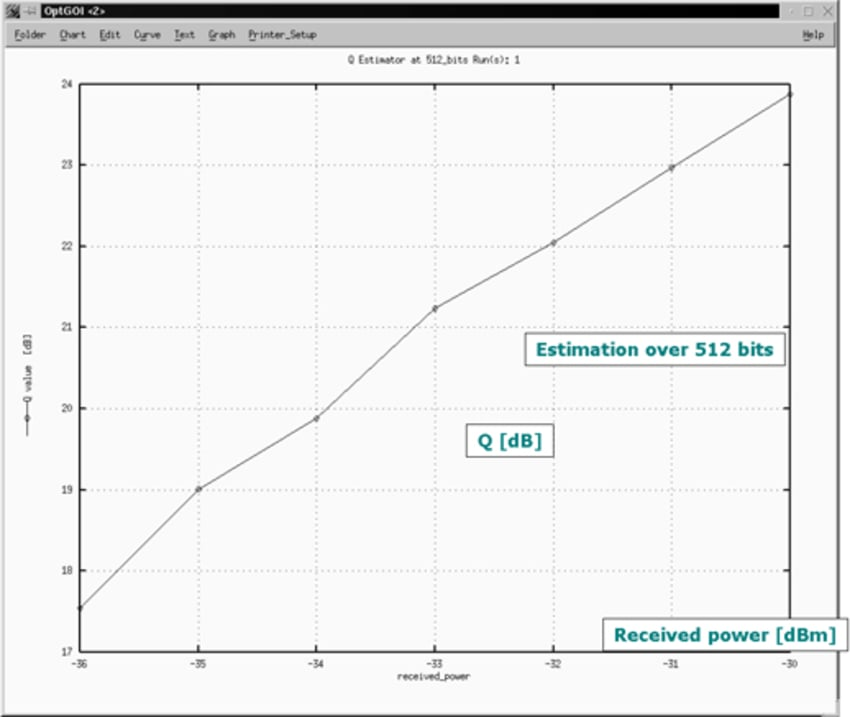This behavior is due to statistical fluctuations of the estimate: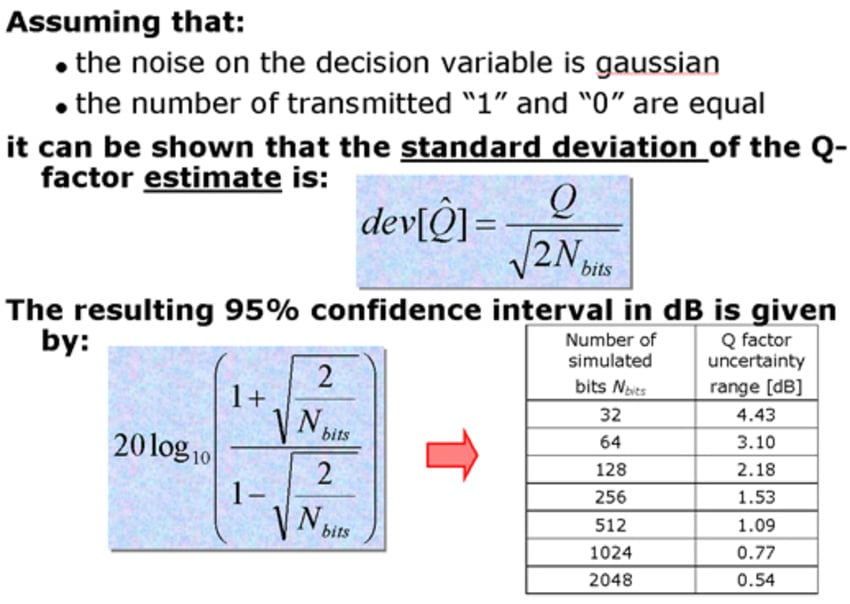The table in above diagram shows that as the number of simulated bits increases, the uncertainty in Q-estimation decreases. For a nominal value of Q = 6 (15.56 dB), the figure below shows "confidence interval" as a function of the number of simulated bits: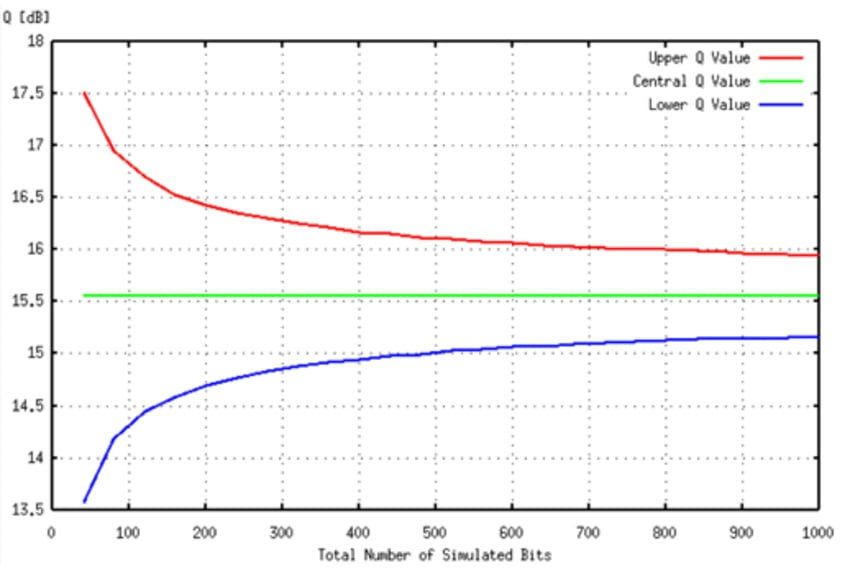The corresponding nominal BER is 1.0E-09 and its fluctuations are shown in the following figure: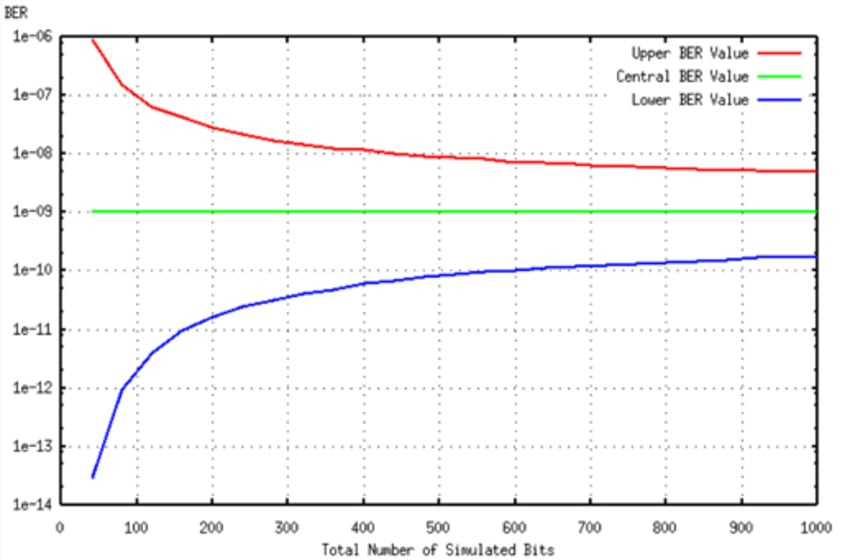We run several simulations by changing the global random generator seed and the attenuation at the receiver. The following two graphs shows the measured Q-factor and BER vs. the received power. Each plot refers to different seeds.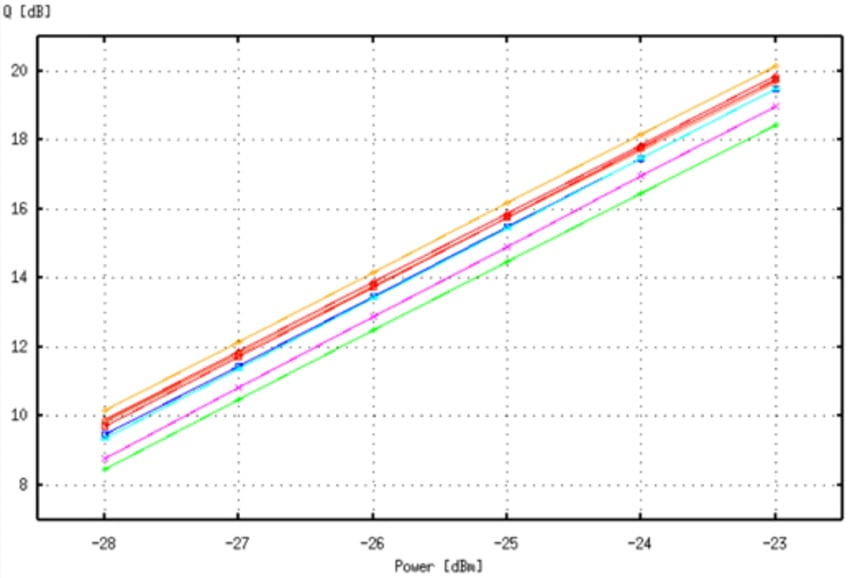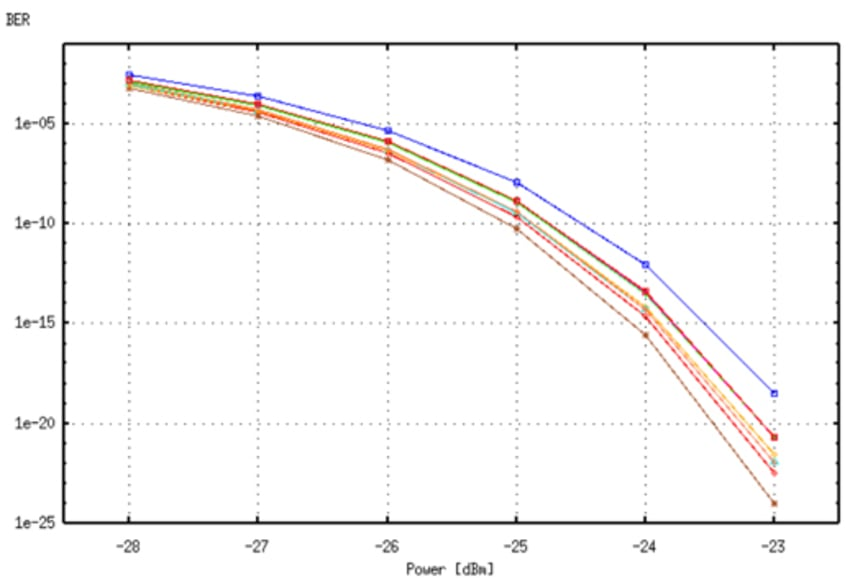We saw that:

1. the Q-factor and BER have an intrinsic uncertainty that decrease while increasing the number of the simulated bits;
2. at least some hundreds of bit should be simulated in order to have sufficiently accurate measurement.

The user should always be aware of these results. Let's suppose that one wants to measure the BER as a function of some given system parameters as, for example, the receiver attenuation. Two situations are possible:

1. the simulation tools force the pseudorandom noise sources to be the same for all simulation runs on the different values of the variable parameters;
2. the simulation tools generate independent pseudorandom noise sources for the different runs.

The resulting uncertainty range is exactly the SAME for both the strategies. The second strategy is the one that OptSim follows in the most of the cases while simulating multiple runs since it avoids to create unwanted correlation between different realization of the pseudorandom sequences. The user should anyway be aware that, for each value of the free parameters in the multiple runs, different noise sequences are generated, and thus the resulting Q or BER can have different positions inside their uncertainty range. The resulting plots, in the same situation of the last graph, but with only one common random generator seed will typically look as the solid line in the graph of the following figure: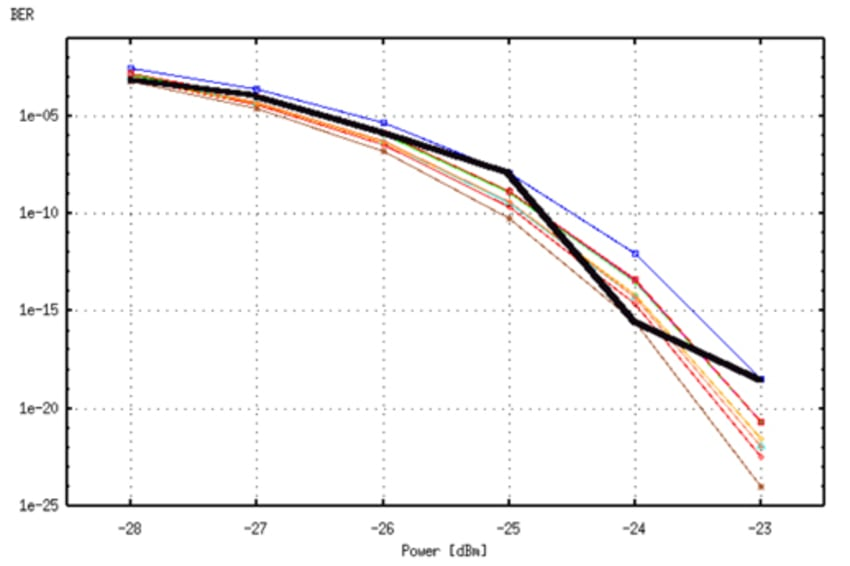The solid line above shows a typical result obtained when the pseudorandom sources are independent from one run to the next in a multiple run simulation.